# Series- Test 3

1)

Find the missing figure in the series from the given answer figures.

[SSC CGL, 2007]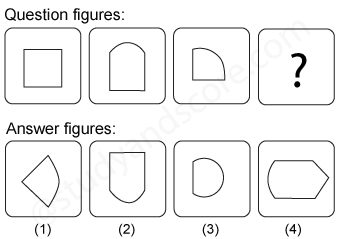2)

Find the missing figure in the series from the given answer figures.

[SSC CGL, 2007]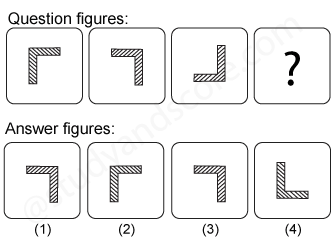3)

Find the missing figure in the series from the given answer figures.

[SSC CGL, 2007]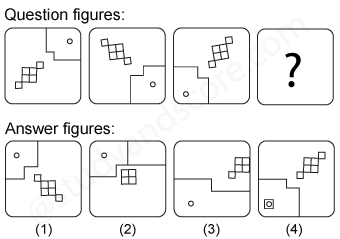4)

Find the missing figure in the series from the given answer figures.

[SSC CGL, 2007]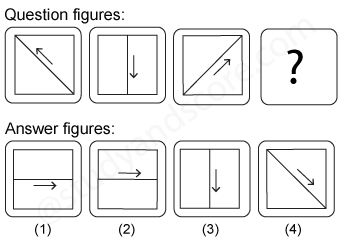5)

Find the missing figure in the series from the given answer figures.

[SSC CGL, 2008]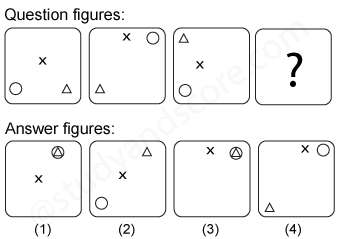--Share with your friends --